SBOS377L October   2006  – January 2020

PRODUCTION DATA.

1. Features
2. Applications
3. Description
1.     Device Images
4. Revision History
5. Pin Configuration and Functions
6. Specifications
7. Detailed Description
1. 7.1 Overview
2. 7.2 Functional Block Diagram
3. 7.3 Feature Description
4. 7.4 Device Functional Modes
8. Application and Implementation
1. 8.1 Application Information
2. 8.2 Typical Application
9. Power Supply Recommendations
10. 10Layout
1. 10.1 Layout Guidelines
2. 10.2 Layout Example
11. 11Device and Documentation Support
1. 11.1 Device Support
1. 11.1.1 Development Support
2. 11.2 Documentation Support
3. 11.3 Related Links
4. 11.4 Receiving Notification of Documentation Updates
5. 11.5 Support Resources
6. 11.6 Trademarks
7. 11.7 Electrostatic Discharge Caution
8. 11.8 Glossary
12. 12Mechanical, Packaging, and Orderable Information

• DRG|8
• DDA|8
• DRG|8
• DDA|8

### 6.8 Typical Characteristics

at TA = 25°C, VS = ±18 V, and RL = 10 kΩ, unless otherwise noted.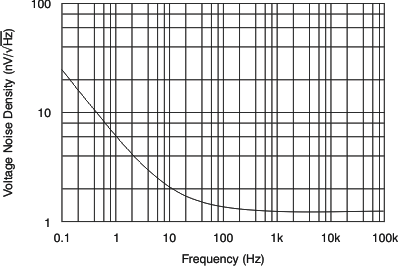Figure 1. Input Voltage Noise Density vs Frequency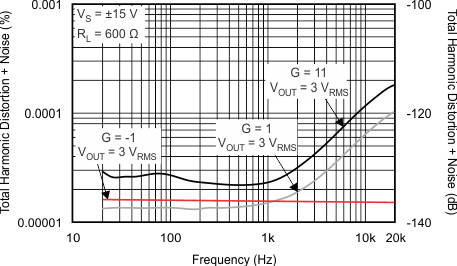Figure 3. THD + N Ratio vs Frequency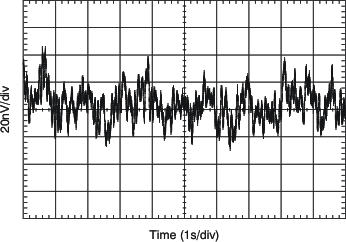Figure 5. 0.1- to 10-Hz Noise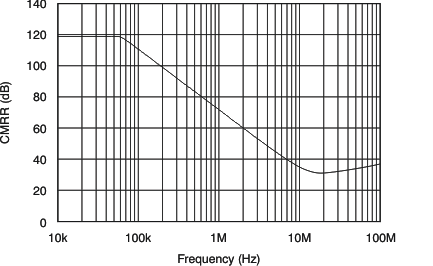Figure 7. Common-Mode Rejection Ratio vs FrequencyFigure 9. Gain and Phase vs Frequency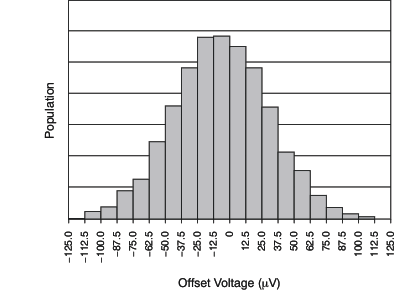Figure 11. Offset Voltage Production Distribution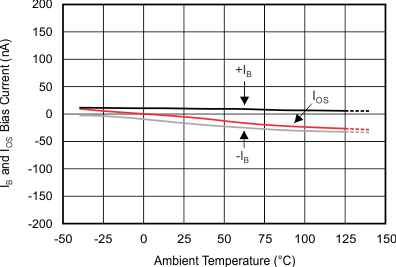Figure 13. IB and IOS Current vs Temperature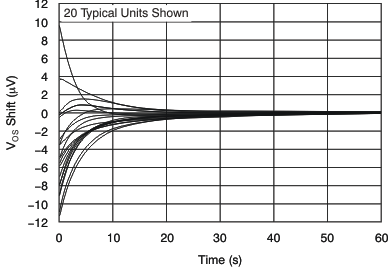Figure 15. VOS Warm-Up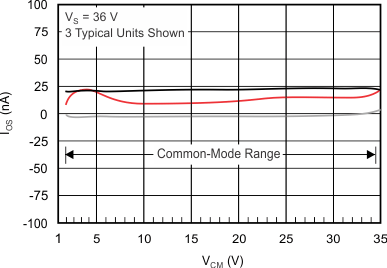Figure 17. Input Offset Current vs Common-Mode Voltage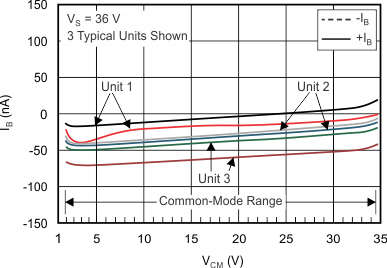Figure 19. Input Bias Current vs Common-Mode Voltage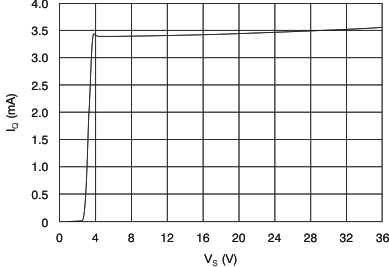Figure 21. Quiescent Current vs Supply Voltage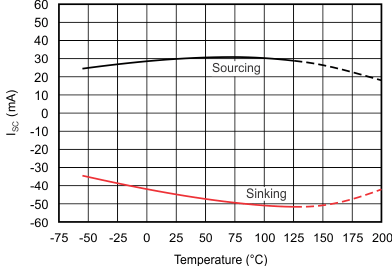Figure 23. Short-Circuit Current vs Temperature(100 mV)
Figure 25. Small-Signal Step Response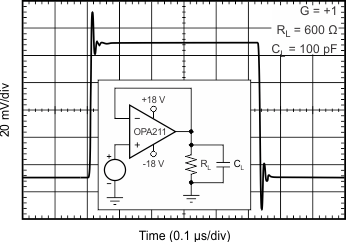(100 mV)
Figure 27. Small-Signal Step Response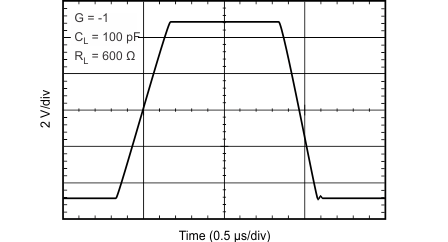Figure 29. Large-Signal Step Response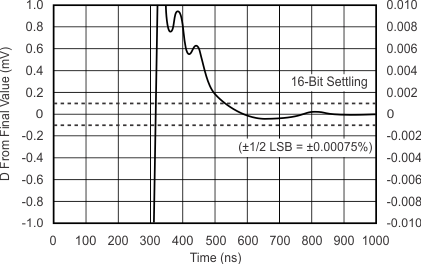10 VPP CL = 100 pF
Figure 31. Large-Signal Positive Settling Time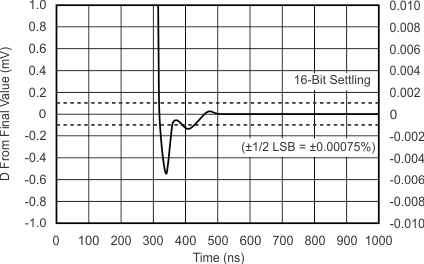10 VPP CL = 100 pF
Figure 33. Large-Signal Negative Settling Time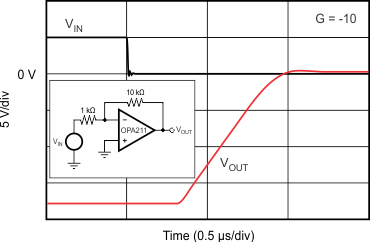Figure 35. Negative Overload Recovery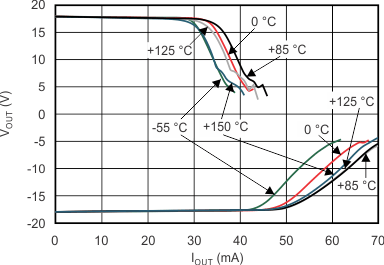Figure 37. Output Voltage vs Output CurrentFigure 39. Turnoff Transient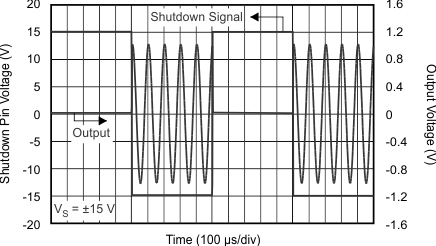Figure 41. Turnon and Turnoff Transient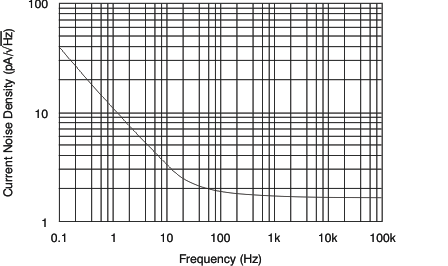Figure 2. Input Current Noise Density vs FrequencyFigure 4. THD + N Ratio vs Output Voltage Amplitude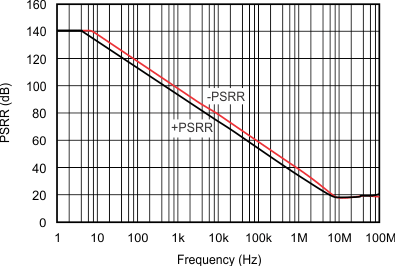Figure 6. Power-Supply Rejection Ratio vs Frequency (Referred to Input)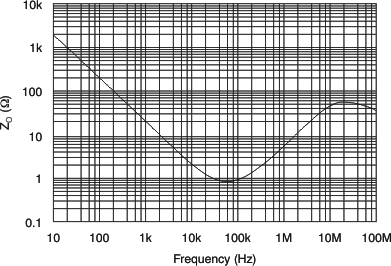Figure 8. Open-Loop Output Impedance vs Frequency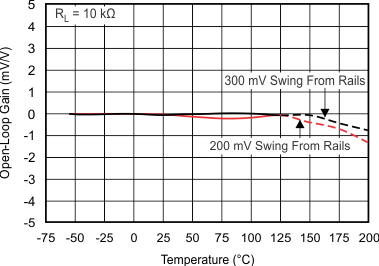Figure 10. Open-Loop Gain vs Temperature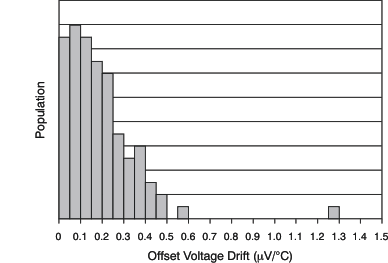Figure 12. Offset Voltage Drift Production Distribution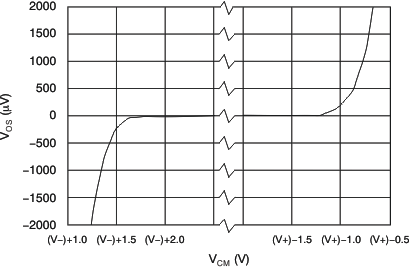Figure 14. Offset Voltage vs Common-Mode Voltage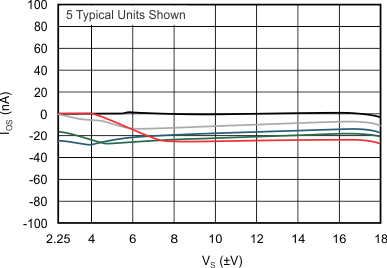Figure 16. Input Offset Current vs Supply Voltage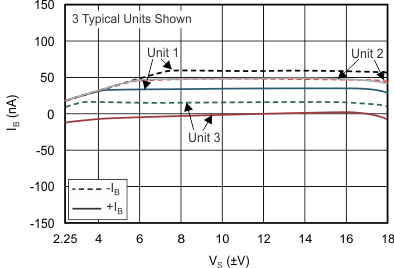Figure 18. Input Bias Current vs Supply Voltage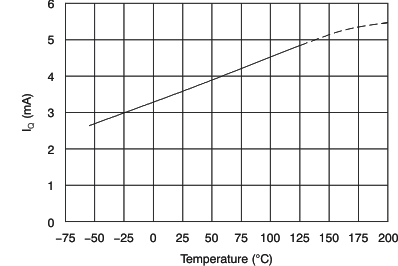Figure 20. Quiescent Current vs Temperature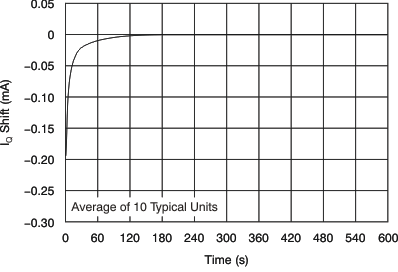Figure 22. Normalized Quiescent Current vs Time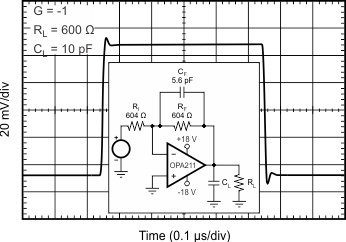Figure 24. Small-Signal Step Response (100 mV)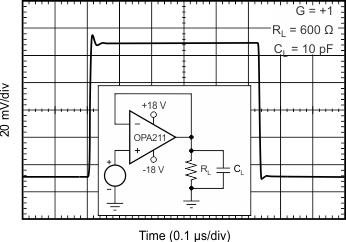(100 mV)
Figure 26. Small-Signal Step Response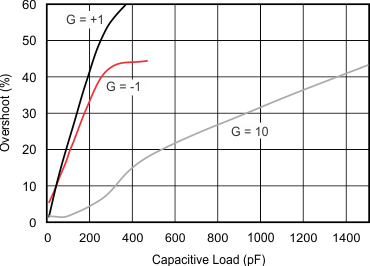(100-mV output step)
Figure 28. Small-Signal Overshoot vs Capacitive LoadFigure 30. Large-Signal Step Response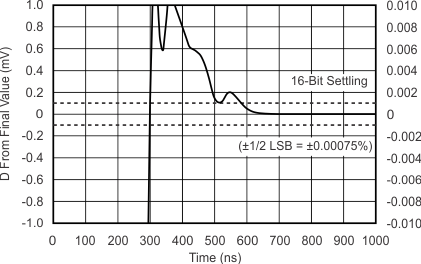10 VPP CL = 10 pF
Figure 32. Large-Signal Positive Settling Time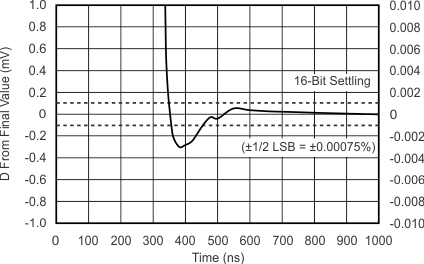10 VPP CL = 10 pF
Figure 34. Large-Signal Negative Settling Time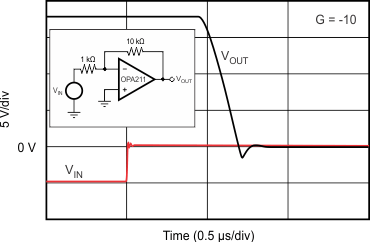Figure 36. Positive Overload Recovery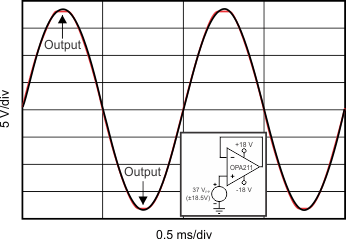Figure 38. No Phase Reversal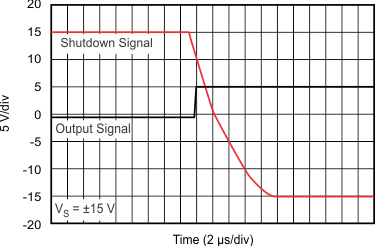Figure 40. Turnon Transient